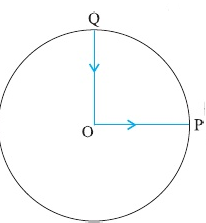Question

Solution

Correct option is

58 m.

Hence h is given bySimplifying and putting g = 10 ms–2, we getThe positive roof of this quadratic gives h = 58 m.

SIMILAR QUESTIONS

Q1

A stone ‘A’ is dropped from the top of a tower 20 m high simultaneously another stone ‘B’ is thrown up from the bottom of the tower so that it can reach just on the top of tower. What is the distance of the stones from the ground while they pass each other.

Q2A cyclist starts from the centre O of a circular track of radius r = 1 km, reaches the edge P of the track and then cycles along circumference and stops at point Q. if the total time take is 10 min, what is the average velocity of the cyclist?

Q3

Give the speed-time graph of the motion of a car. What is the ratio of the distance travelled by the during the last two seconds to the total distance travelled in seven seconds?